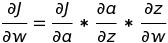Related Articles

# Implementation of Logistic Regression from Scratch using Python

• Last Updated : 25 Oct, 2020

#### Introduction:

Logistic Regression is a supervised learning algorithm that is used when the target variable is categorical. Hypothetical function h(x) of linear regression predicts unbounded values. But in the case of Logistic Regression, where the target variable is categorical we have to strict the range of predicted values. Consider a classification problem, where we need to classify whether an email is a spam or not. So, the hypothetical function of linear regression could not be used here to predict as it predicts unbound values, but we have to predict either 0 or 1.

To do, so we apply the sigmoid activation function on the hypothetical function of linear regression. So the resultant hypothetical function for logistic regression is given below :

```h( x ) = sigmoid( wx + b )

Here, w is the weight vector.
x is the feature vector.
b is the bias.

sigmoid( z ) = 1 / ( 1 + e( - z ) )
```

#### Mathematical Intuition:

The cost function of linear regression ( or mean square error ) can’t be used in logistic regression because it is a non-convex function of weights. Optimizing algorithms like i.e gradient descent only converge convex function into a global minimum.

So, the simplified cost function we use :

```J = - ylog( h(x) ) - ( 1 - y )log( 1 - h(x) )

here, y is the real target value

h( x ) = sigmoid( wx + b )

For y = 0,

J = - log( 1 - h(x) )

and y = 1,

J = - log( h(x) )
```

This cost function is because when we train, we need to maximize the probability by minimizing the loss function.

```repeat until convergence  {
tmpi = wi - alpha * dwi
wi = tmpi
}
where alpha is the learning rate.
```

The chain rule is used to calculate the gradients like i.e dw.Chain rule for dw

```here, a = sigmoid( z ) and z = wx + b.
```

#### Implementation:

It has 8 features columns like i.e “Age“, “Glucose” e.t.c, and the target variable “Outcome” for 108 patients. So in this, we will train a Logistic Regression Classifier model to predict the presence of diabetes or not for patients with such information.

 `# Importing libraries``import` `numpy as np``import` `pandas as pd``from` `sklearn.model_selection ``import` `train_test_split``import` `warnings``warnings.filterwarnings( ``"ignore"` `)`` ` `# to compare our model's accuracy with sklearn model``from` `sklearn.linear_model ``import` `LogisticRegression``# Logistic Regression``class` `LogitRegression() :``    ``def` `__init__( ``self``, learning_rate, iterations ) :        ``        ``self``.learning_rate ``=` `learning_rate        ``        ``self``.iterations ``=` `iterations``         ` `    ``# Function for model training    ``    ``def` `fit( ``self``, X, Y ) :        ``        ``# no_of_training_examples, no_of_features        ``        ``self``.m, ``self``.n ``=` `X.shape        ``        ``# weight initialization        ``        ``self``.W ``=` `np.zeros( ``self``.n )        ``        ``self``.b ``=` `0`        `        ``self``.X ``=` `X        ``        ``self``.Y ``=` `Y``         ` `        ``# gradient descent learning``                 ` `        ``for` `i ``in` `range``( ``self``.iterations ) :            ``            ``self``.update_weights()            ``        ``return` `self``     ` `    ``# Helper function to update weights in gradient descent``     ` `    ``def` `update_weights( ``self` `) :           ``        ``A ``=` `1` `/` `( ``1` `+` `np.exp( ``-` `( ``self``.X.dot( ``self``.W ) ``+` `self``.b ) ) )``         ` `        ``# calculate gradients        ``        ``tmp ``=` `( A ``-` `self``.Y.T )        ``        ``tmp ``=` `np.reshape( tmp, ``self``.m )        ``        ``dW ``=` `np.dot( ``self``.X.T, tmp ) ``/` `self``.m         ``        ``db ``=` `np.``sum``( tmp ) ``/` `self``.m ``         ` `        ``# update weights    ``        ``self``.W ``=` `self``.W ``-` `self``.learning_rate ``*` `dW    ``        ``self``.b ``=` `self``.b ``-` `self``.learning_rate ``*` `db``         ` `        ``return` `self``     ` `    ``# Hypothetical function  h( x ) ``     ` `    ``def` `predict( ``self``, X ) :    ``        ``Z ``=` `1` `/` `( ``1` `+` `np.exp( ``-` `( X.dot( ``self``.W ) ``+` `self``.b ) ) )        ``        ``Y ``=` `np.where( Z > ``0.5``, ``1``, ``0` `)        ``        ``return` `Y`` ` ` ` `# Driver code`` ` `def` `main() :``     ` `    ``# Importing dataset    ``    ``df ``=` `pd.read_csv( ``"diabetes.csv"` `)``    ``X ``=` `df.iloc[:,:``-``1``].values``    ``Y ``=` `df.iloc[:,``-``1``:].values``     ` `    ``# Splitting dataset into train and test set``    ``X_train, X_test, Y_train, Y_test ``=` `train_test_split(``      ``X, Y, test_size ``=` `1``/``3``, random_state ``=` `0` `)``     ` `    ``# Model training    ``    ``model ``=` `LogitRegression( learning_rate ``=` `0.01``, iterations ``=` `1000` `)``     ` `    ``model.fit( X_train, Y_train )    ``    ``model1 ``=` `LogisticRegression()    ``    ``model1.fit( X_train, Y_train)``     ` `    ``# Prediction on test set``    ``Y_pred ``=` `model.predict( X_test )    ``    ``Y_pred1 ``=` `model1.predict( X_test )``     ` `    ``# measure performance    ``    ``correctly_classified ``=` `0`    `    ``correctly_classified1 ``=` `0``     ` `    ``# counter    ``    ``count ``=` `0`    `    ``for` `count ``in` `range``( np.size( Y_pred ) ) :  ``       ` `        ``if` `Y_test[count] ``=``=` `Y_pred[count] :            ``            ``correctly_classified ``=` `correctly_classified ``+` `1``         ` `        ``if` `Y_test[count] ``=``=` `Y_pred1[count] :            ``            ``correctly_classified1 ``=` `correctly_classified1 ``+` `1``             ` `        ``count ``=` `count ``+` `1``         ` `    ``print``( ``"Accuracy on test set by our model       :  "``, ( ``      ``correctly_classified ``/` `count ) ``*` `100` `)``    ``print``( ``"Accuracy on test set by sklearn model   :  "``, ( ``      ``correctly_classified1 ``/` `count ) ``*` `100` `)`` ` ` ` `if` `__name__ ``=``=` `"__main__"` `:     ``    ``main()`

#### Output :

```Accuracy on test set by our model       :   58.333333333333336
Accuracy on test set by sklearn model   :   61.111111111111114
```

Note: The above-trained model is to implement the mathematical intuition not just for improving accuracies.

Attention reader! Don’t stop learning now. Get hold of all the important Machine Learning Concepts with the Machine Learning Foundation Course at a student-friendly price and become industry ready.

My Personal Notes arrow_drop_up